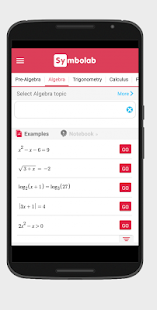## Recommended Posts

Symbolab - Math solver v5.3.0 [Pro]
Requirements: Android 4.4+
Overview: Your private math tutor, solves any math problem with steps!Your private math tutor, solves any math problem with steps! equations, integrals, derivatives, , limits and much more.
(Steps require a one-time in-app purchase)

Symbolab Math Solver app is composed of over a hundred Symbolab most powerful calculators:
Equation Calculator
Integral Calculator
Derivative Calculator
Limit calculator
Inequality Calculator
Trigonometry Calculator
Matrix Calculator
Functions Calculator
Series Calculator
ODE Calculator
Laplace Transform Calculator

Symbolab Math Solver solves any math problem including Pre- Algebra, Algebra, Pre-Calculus, Calculus, Trigonometry, Functions, Matrix, Vectors, Geometry and Statistics.

What's New:
* Added new subjects (Limit of Sums, Multi-var Limits, Derivative Using the Definition, Complex Equations, Trig Inequalities, and more)
* Enhancements and bug fixes

`https://play.google.com/store/apps/details?id=com.devsense.symbolab`

#### Archived

This topic is now archived and is closed to further replies.

• ### Similar Content

• 0
• 0
• 0
• 0
• 0
• 0
• 0
• 0
• 0
• 0
• 0
• 0
• 0
• 0
• 0
×
×
• Create New...
PixFuture exclusive partner.